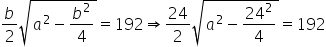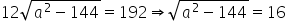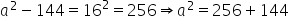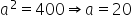Maths-
General
Easy

Question

# The base of an isosceles triangle is 24 cm and its area is 192 cm2 . Find its perimeterHint:

## The correct answer is: 64 cm

### It is given that base, b = 24 cm andArea of an isosceles triangle = 192 cm2So, length of two equal sides = 20 cmNow, perimeter of the triangle = sum of all sides= 20 + 20 + 24 = 64 cm#### With Turito Foundation.#### Get an Expert Advice From Turito.